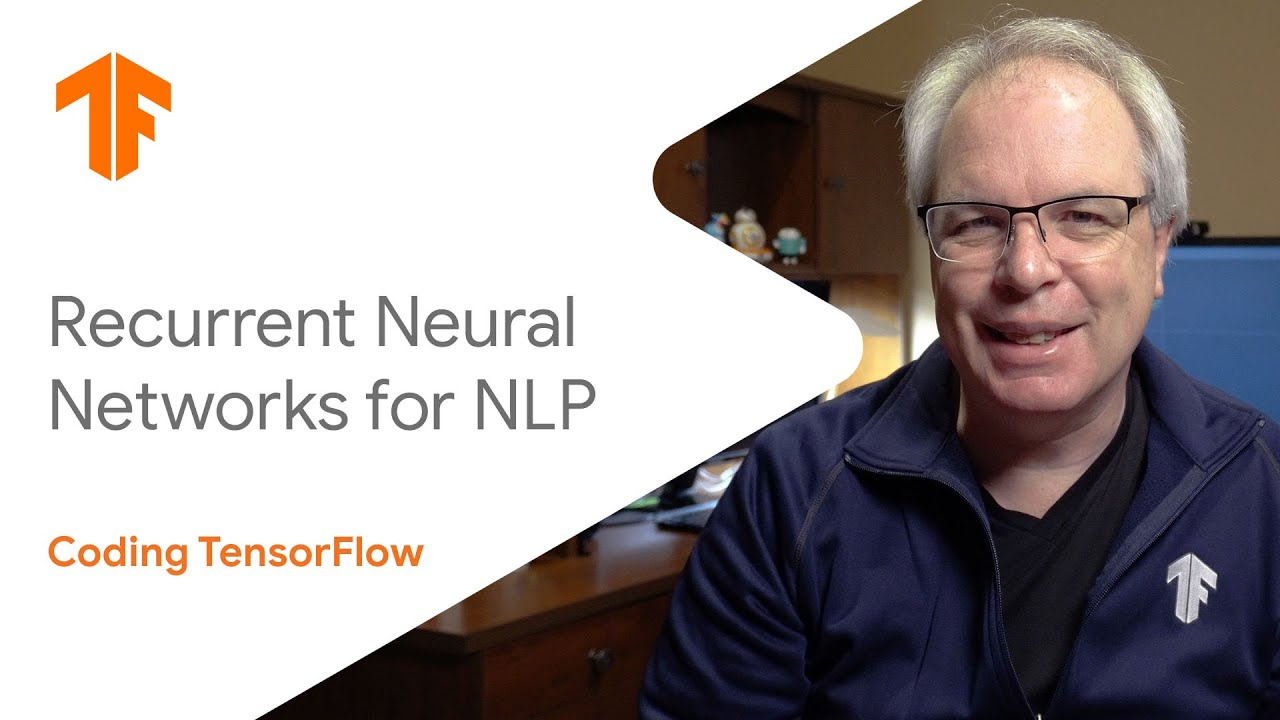# ML with Recurrent Neural NetworksWelcome to this episode in Natural Language Processing Zero to Hero with TensorFlow. In the previous videos in this series you saw how to tokenize text.

Welcome to this episode in Natural Language Processing Zero to Hero with TensorFlow. In the previous videos in this series you saw how to tokenize text, and use sequences of tokens to train a neural network. In the next videos we’ll look at how neural networks can generate text and even write poetry, beginning with an introduction to Recurrent Neural Networks (RNNs).

## Neural network: what is a neural network?

Neural networks, as their name implies, are computer algorithms modeled after networks of neurons in the human brain. Learn more about neural networks from Algorithmia.

## A Comparative Analysis of Recurrent Neural Networks

Recurrent neural networks, also known as RNNs, are a class of neural networks that allow previous outputs to be used as inputs while having hidden states.

## Recurrent Neural Networks for Multilabel Text Classification Tasks

The purpose of this project is to build and evaluate Recurrent Neural Networks(RNNs) for sentence-level classification tasks. Let's understand about recurrent neural networks for multilabel text classification tasks.

## Neural Network on Beer Dataset

Artificial neural networks (ANNs), usually simply called neural networks (NNs), are computing systems vaguely inspired by the biological neural networks that constitute animal brains.

## Convolutional Neural Network: How is it different from the other networks?

Convolutional Neural Network: How is it different from the other networks? What’s so unique about CNNs and what does convolution really do? This is a math-free introduction to the wonders of CNNs.